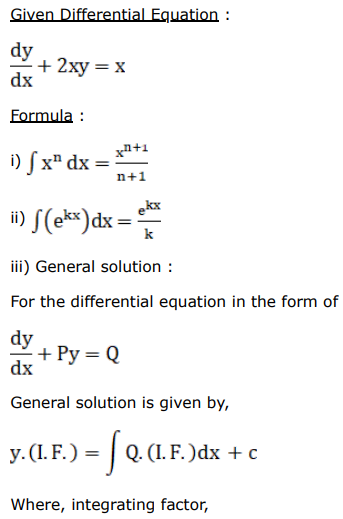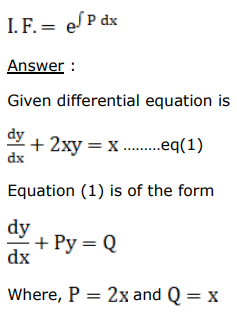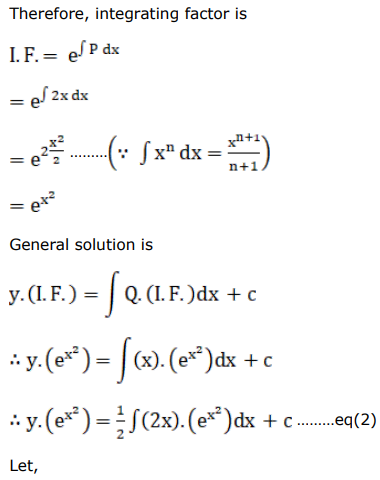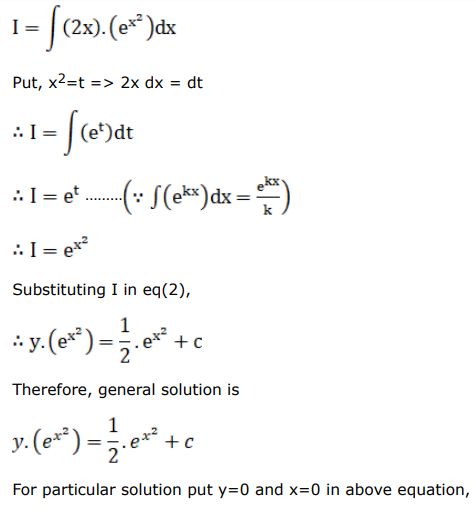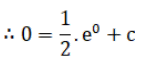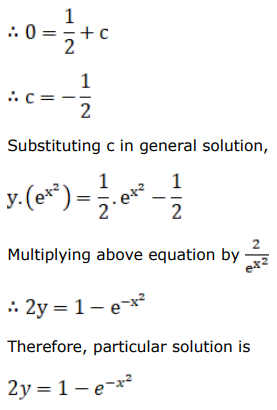# Find a particular solution satisfying the given condition for each of the following differential equations.Question:

Find a particular solution satisfying the given condition for each of the following differential equations.

$\frac{\mathrm{dy}}{\mathrm{dx}}+2 \mathrm{xy}=\mathrm{x}$, given that $y=0$ when $x=0$

Solution: